Browse by Topic
Related Topics

# Splines Come to Mathematica

One of the areas I contributed to Mathematica 7 was support for splines. The word “spline” originated from the term used by ship builders referring to thin wood pieces.

Over the last 40 years, splines have become very popular in computer graphics, computer animation and computer-aided design fields. From containers for household goods to state-of-the-art airplanes, it is hard to find any industrial product without spline surfaces. Also, they are widely used in other mathematical studies, such as interpolation and approximation.

Through its integration of numerics, symbolics and graphics, Mathematica has the opportunity to go much further with splines than has ever been possible before. Mathematica has had basic spline packages for a long time. But in Mathematica 7 we decided to make highly general spline support a core feature of the system.

Splines give another way to represent classes of functions. For decades, mathematicians had been using polynomials for numerical analysis. In the early 20th century, with advances in approximation theory, spline functions were beginning to emerge. The basic idea is simple. In essence, they consist of piecewise polynomials with local supports.

Since Version 5.1, Mathematica has offered general support for piecewise functions, both numerically and symbolically. In Mathematica 7, the B-spline functions can be expanded using PiecewiseExpand. For example, a uniform cubic B-spline basis function can be expanded to the following.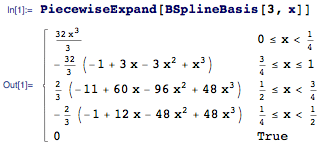Splines have many attractive properties for interpolation. For instance, you can achieve good smoothness without relying on high degrees. Also, it is easier to avoid undesirable effects, such as Runge’s phenomenon. Ever since Mathematica 2, we’ve had the concept of InterpolatingFunction, which allows us to have a symbolic representation for an approximated function defined by interpolation. In Mathematica 7, the spline method is fully integrated in Interpolation. You can easily compare the results of the old "Hermite" method and the new "Spline" method.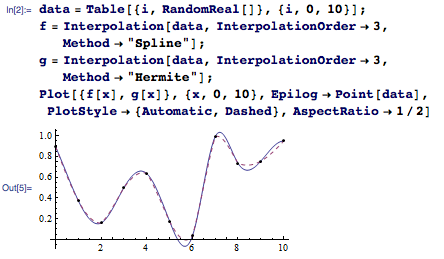In the late 1950s, two French mathematicians, Pierre Bézier from Renault and Paul de Casteljau from Citroën, independently developed spline-based computer-aided design systems for the automakers. Afterward, the popularity of splines literally exploded, and the rest is history.

Before we get into the fun side of splines, let’s discuss basis functions of B-splines, from which the B of B-splines comes. With interactive capability introduced in Mathematica 6, Mathematica is an incredible tool to explore the properties of the spline functions. For instance, a basis function can be created by adding of two lower-degree splines, as illustrated here using Manipulate.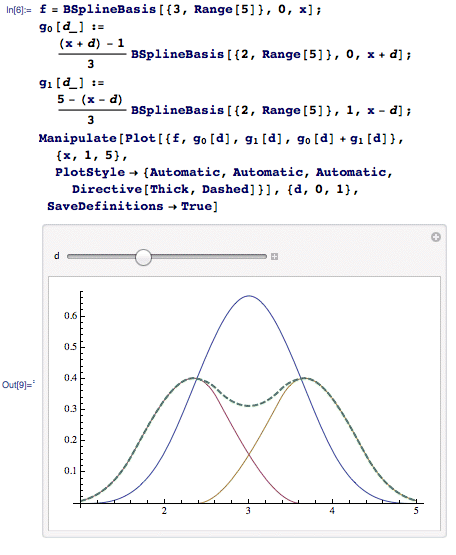In the preceding examples, each piecewise polynomial is defined on an interval with the same length and thus forms a “uniform” basis. However, by using intervals with different lengths, one can generate a family of nonuniform basis functions. The unions of the endpoints of intervals are called “knots.” The following example shows how the knot change affects the cubic basis functions.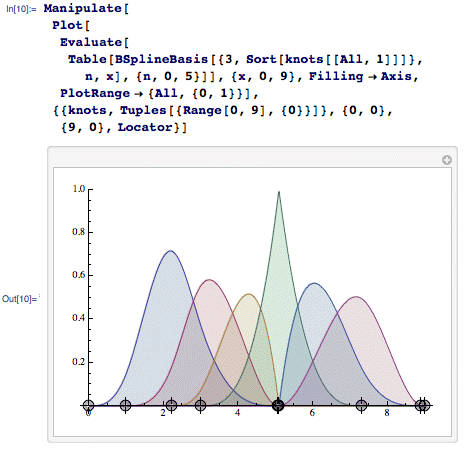From these univariate functions, we can build up multidimensional functions using the tensor products. For instance, a basis function in 2D can be defined by multiplying two univariate functions.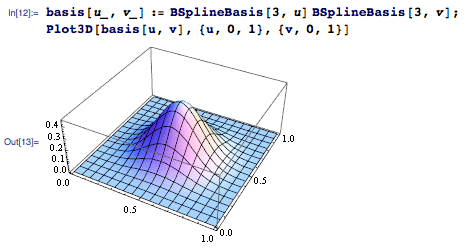Or you can generate a family of 2D functions using different bases.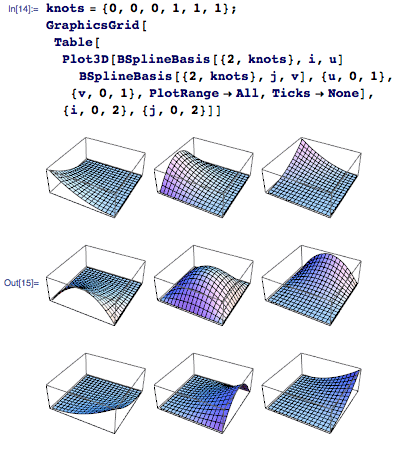The Wolfram Demonstrations Project already has a couple of interesting Demonstrations regarding B-spline basis. “B-Spline Basis Functions” is one such example.

Now, let’s move toward more interesting topics. We will start with curves. B-spline curves can be formulated as a linear summation of points and univariate basis functions.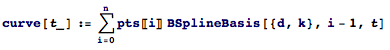And the formula can be further simplified by using Dot. The points are traditionally called “control points,” and they define the shape of the curve in the range space. This is a typical example of a cubic B-spline curve in 2D.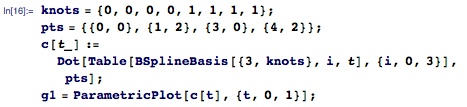But all those indices and dot products can be frustrating. As an alternative, Mathematica 7 supplies a new function, called BSplineFunction, which makes the construction much simpler.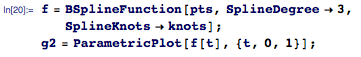It is not just about the convenience. Internally, when BSplineFunction is called, Mathematica prepares important parameters that make overall computation much faster in subsequent calls.

But we haven’t stopped here. Mathematica 7 also provides new B-spline graphics primitives, such as BSplineCurve and BSplineSurface. The preceding example can be written purely in graphics language.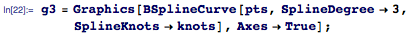And you can see that all three approaches result in identical curves.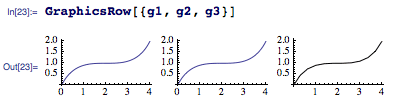The new features in Mathematica provide very detailed controls over B-splines. We can deal with arbitrary degrees and arbitrary knot configurations. I made a curve editor based on a Demonstration I wrote before Mathematica 7. This example allows you to control every aspect of B-spline curves, as well as the ability to inspect their basis functions.

In addition, SplineWeights are provided for rational B-splines, or NURBS. With rational splines, one can create shapes that are not possible to represent with polynomial—for instance, a perfect circle: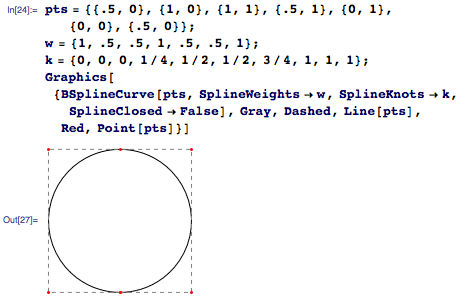B-spline surfaces can be created in the same fashion, except that now we need to use tensor products. Instead of a list of points, we need to use a matrix of points, which is sometimes called a control mesh. The following example shows a simple B-spline surface using BSplineSurface.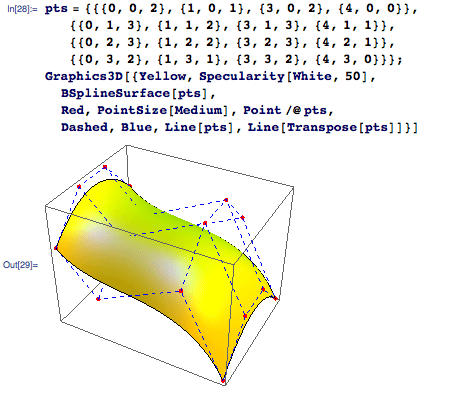Of course, one can use BSplineFunction with ParametricPlot3D instead. Naturally, all the options for ParametricPlot3D work perfectly well with B-spline surfaces.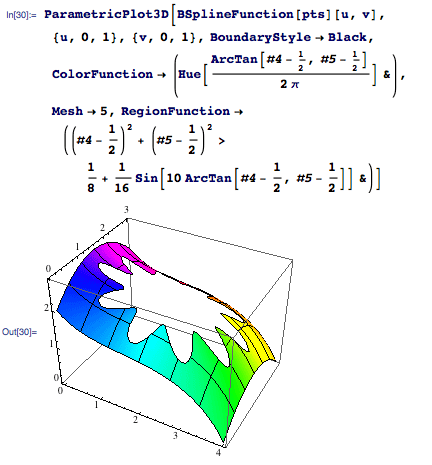As I mentioned, splines surfaces have been used in car design for almost half a century. I wrote this little Demonstration to illustrate how a boxy design can be transformed into a futuristic body, by increasing spline degrees. Of course, this Demonstration is just for fun, and does not reflect real-world car design (which is an incredibly elaborate task, by the way).

The new spline functionality in Mathematica 7 is very rich and general. For instance, one can easily make a 4D spline manifold (or any number of dimensions, for that matter) using BSplineFunction.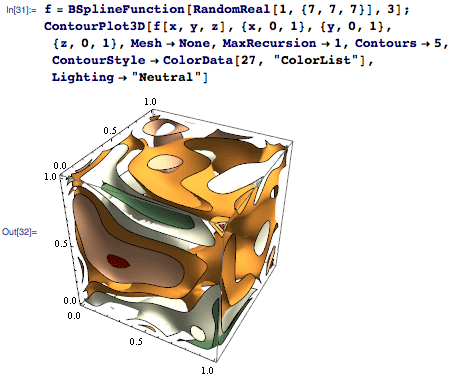I will continue the story about splines in Mathematica 7 in a later post. I would like to discuss a few Demonstrations that I wrote specifically for the B-splines, and some other features that I haven’t mentioned here. It will be more interesting (and practical), so please stay tuned.